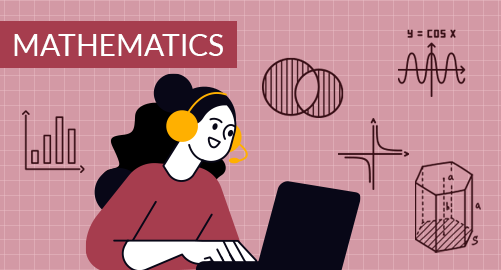#### through a demo today# Year 9 Mathematics

Mathematics
Year 9

The Year 9 Mathematics curriculum extends deeper into the field of application, providing students with diverse skills and information necessary for both academic progress and practical approach. The topics include algebra, financial mathematics, geometry, coordinate geometry, measurement, trigonometry, data analysis and probability.

## What you'll learn

• 1

Basic Algebra

• 2

Equations and Inequations

• 3

Financial Mathematics

• 4

Geometry

• 5

Coordinate Geometry

• 6

Measurement

• 7

Trigonometry

• 8

Data Analysis

• 9

Probability

## Skills you'll learnAlgebraic Proficiency Skills: Numerical skills, algebraic manipulation, polynomial operations, exponent handling, factorisation techniques, pattern recognitionProblem-Solving Skills: Solving linear equations and inequations, a system of linear equations, quadratic equations proficiency, quadratic equations graphing, algebraic manipulationFinancial Skills: Working with percentages; finding profit, loss and discounts; understanding tax basics, interest types and depreciation; tax and interest calculationsGeometrical Skills and Spatial Reasoning: Proficiency in measuring angles, understanding and identifying congruent and similar figures, applying transformations, quadrilateral expertise, special quadrilateral tests, enlargement techniques, area of similar figures calculationsCoordinate Geometry Competencies: Locating coordinates, calculating distance and midpoint of an interval, understanding and finding gradient, formulating equations for lines and circles, graphing lines and circlesMeasurement Proficiency and Conversion Skills: 2D shape measurement, 3D shape measurement, unit conversion, measurement units, time calculationsCritical Thinking Skills: Applying Pythagoras theorem and its converse, trigonometric ratios understanding, complementary angles, bearing interpretationData Analysis and Interpretation: Data organisation, representing data, interpreting data, finding the central value of data, statistical data investigation processPrediction Skills: Understanding probability and related terms, event and outcome analysis, set operations in probability, estimating probability, handling multiple events, sampling with replacement